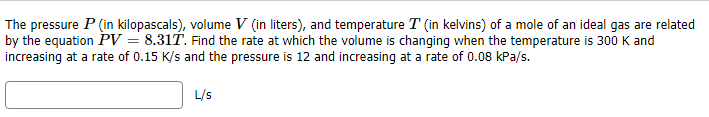# The pressure P (in kilopascals), volume V (in liters), and temperature T (in kelvins) of a mole of an ideal gas are relatedby the equation PV = 8.31T. Find the rate at which the volume is chanqing when the temperature is 300 K andincreasing at a rate of 0.15 K/s and the pressure is 12 and increasing at a rate of 0.08 kPa/s.L/s

Question
10 viewshelp_outlineImage TranscriptioncloseThe pressure P (in kilopascals), volume V (in liters), and temperature T (in kelvins) of a mole of an ideal gas are related by the equation PV = 8.31T. Find the rate at which the volume is chanqing when the temperature is 300 K and increasing at a rate of 0.15 K/s and the pressure is 12 and increasing at a rate of 0.08 kPa/s. L/s fullscreen
check_circle

Step 1

Rate of change of a functi...

### Want to see the full answer?

See Solution

#### Want to see this answer and more?

Solutions are written by subject experts who are available 24/7. Questions are typically answered within 1 hour.*

See Solution
*Response times may vary by subject and question.
Tagged in

### Derivative